## 电子科技大学《高等数学(理科本科)》20春期末考试

------------------------
1.9. 不定积分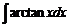对应的分部积分公式可表示为（ ）
A.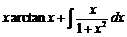B.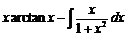C.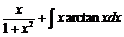D.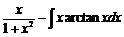2.11. 函数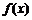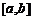连续，下列等式正确的是（ ）
A.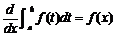B.C.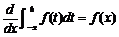D.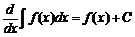3.15. 定积分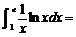（ ）
A.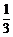B.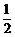C.2
D.3

4.4. 由分项积分法，不定积分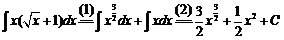A.第（1）步正确，第（2）步不正确
B.第（1）步正确，第（2）步也正确
C.第（1）步不正确，第（2）步正确
D.第（1）步不正确，第（2）步也不正确

5.7. 不定积分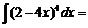（ ）
A.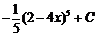B.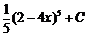C.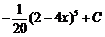D.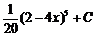6.13. 定积分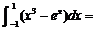（ ）
A.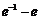B.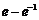C.e
D.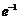7.19. 微分方程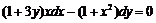的通解是（ ）
A.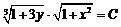B.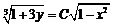C.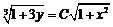D.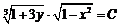8.9. 不定积分（ ）
A.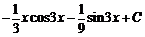B.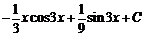C.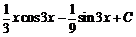D.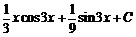9.19. 微分方程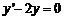的通解是（ ）
A.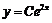B.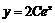C.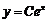D.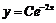10.20. 微分方程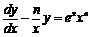的通解是（ ）
A.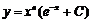B.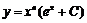C.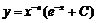D.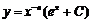11.19. 微分方程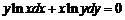的通解是（ ）
A.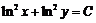B.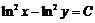C.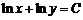D.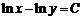12.8. 不定积分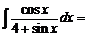（ ）
A.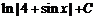B.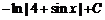C.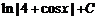D.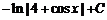13.4. 不定积分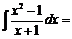（ ）
A.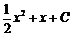B.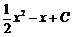C.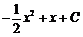D.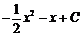14.6. 设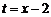，则不定积分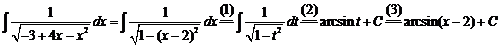A.第（1）步开始出错
B.第（2）步开始出错
C.第（3）步开始出错
D.全部正确

15.7. 不定积分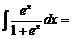（ ）
A.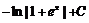B.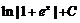C.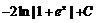D.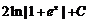16.8. 不定积分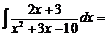（ ）
A.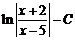B.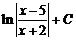C.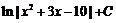D.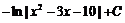17.7. 不定积分（ ）
A.B.C.D.18.19. 微分方程的通解是（ ）
A.B.C.D.19.17. 曲线 直线 及x轴所围成的平面图形的面积，可以表示为（ ）
A.B.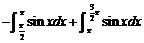C.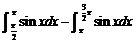D.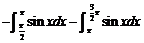20.6. 设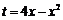，则不定积分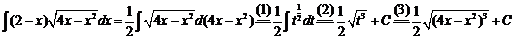A.第（1）步开始出错
B.第（2）步开始出错
C.第（3）步开始出错
D.全部正确